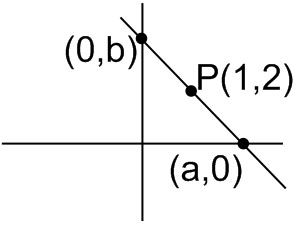#### Let  L  be the line passing through the point P(1, 2) such that its intercepted segment between the co-ordinate axes is bisected at P.  If L1 is the line perpendicular to L and passing through the point (-2, 1), then the point of intersection of L and L1 is : Option 1)Option 2)Option 3)Option 4)As we learnt in

Intercept form of a straight line -- whereinandare the-intercept and-intercept respectively.

Equation of a line perpendicular to a given line -is the line perpendicular to.

- whereinis some other constant  than.

Herehence equation of straight line is(intercept form)LineisPassing through (-2, 1)Option 1)Correct option

Option 2)Incorrect Option

Option 3)Incorrect Option

Option 4)Incorrect Option

## Crack JEE Main with "AI Coach"

• HD Video Lectures
• Unlimited Mock Tests
• Faculty SupportOption no. 1